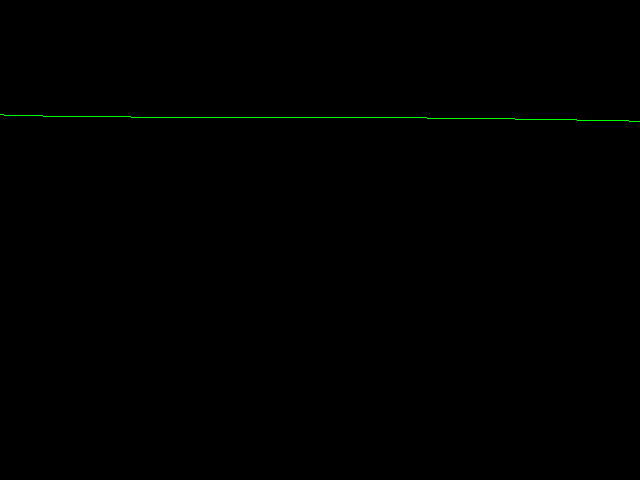NNet is a (metaprogrammed) neural network. It allows the selection of the network layout (number of layers and activation functions) in a simple way, without runtime costs -- check out the following 2 hidden layers network:

``````typedef NeuralNetwork<
float,
boost::mpl::vector<
Layer<Tanh, 1>,
Layer<Tanh, 12>,
Layer<Tanh, 6>,
Layer<Tanh, 1>
>
> NNet;
``````

Sources include a simple SDL example (where the network is trained to learn the blue points), below a sample execution: